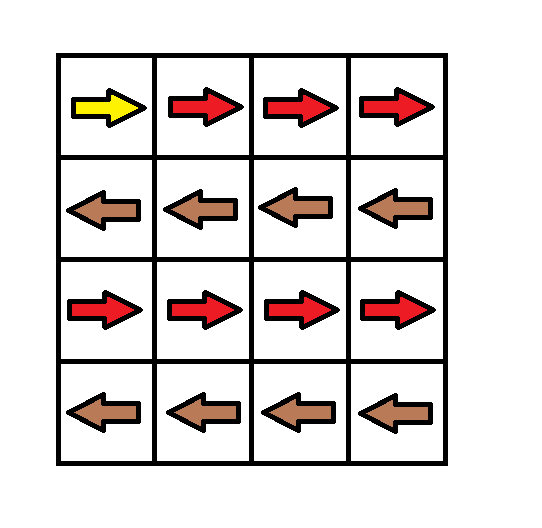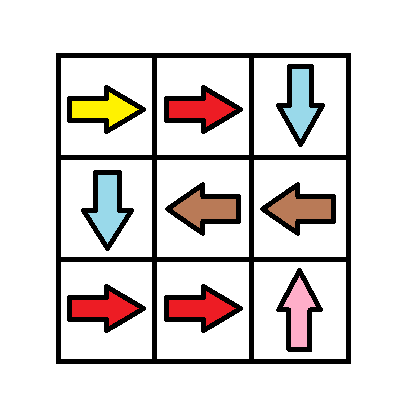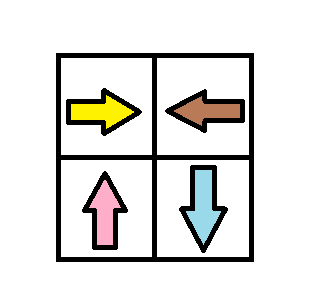# GeetCode Hub

Given a m x n `grid`. Each cell of the `grid` has a sign pointing to the next cell you should visit if you are currently in this cell. The sign of `grid[i][j]` can be:

• 1 which means go to the cell to the right. (i.e go from `grid[i][j]` to `grid[i][j + 1]`)
• 2 which means go to the cell to the left. (i.e go from `grid[i][j]` to `grid[i][j - 1]`)
• 3 which means go to the lower cell. (i.e go from `grid[i][j]` to `grid[i + 1][j]`)
• 4 which means go to the upper cell. (i.e go from `grid[i][j]` to `grid[i - 1][j]`)

Notice that there could be some invalid signs on the cells of the `grid` which points outside the `grid`.

You will initially start at the upper left cell `(0,0)`. A valid path in the grid is a path which starts from the upper left cell `(0,0)` and ends at the bottom-right cell `(m - 1, n - 1)` following the signs on the grid. The valid path doesn't have to be the shortest.

You can modify the sign on a cell with `cost = 1`. You can modify the sign on a cell one time only.

Return the minimum cost to make the grid have at least one valid path.

Example 1:```Input: grid = [[1,1,1,1],[2,2,2,2],[1,1,1,1],[2,2,2,2]]
Output: 3
Explanation: You will start at point (0, 0).
The path to (3, 3) is as follows. (0, 0) --> (0, 1) --> (0, 2) --> (0, 3) change the arrow to down with cost = 1 --> (1, 3) --> (1, 2) --> (1, 1) --> (1, 0) change the arrow to down with cost = 1 --> (2, 0) --> (2, 1) --> (2, 2) --> (2, 3) change the arrow to down with cost = 1 --> (3, 3)
The total cost = 3.
```

Example 2:```Input: grid = [[1,1,3],[3,2,2],[1,1,4]]
Output: 0
Explanation: You can follow the path from (0, 0) to (2, 2).
```

Example 3:```Input: grid = [[1,2],[4,3]]
Output: 1
```

Example 4:

```Input: grid = [[2,2,2],[2,2,2]]
Output: 3
```

Example 5:

```Input: grid = []
Output: 0
```

Constraints:

• `m == grid.length`
• `n == grid[i].length`
• `1 <= m, n <= 100`

class Solution { public int minCost(int[][] grid) { } }